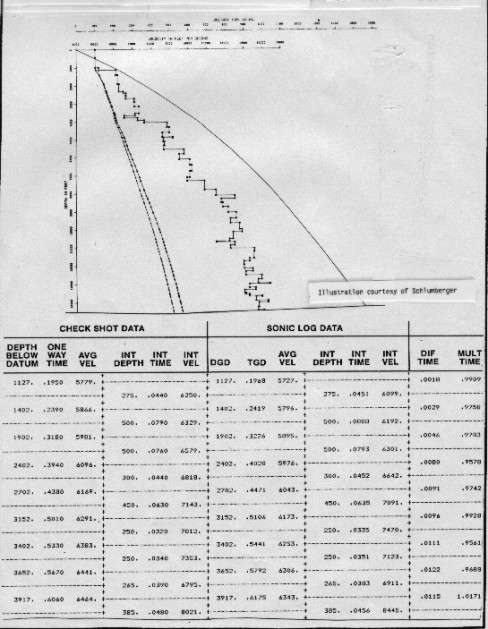Time to Depth Conversions
If we assume that we have a list of seismic times and corresponding stacking velocities (Vstk) from a constant velocity stack, or similarly derived seismic velocity analysis, we can calculate the interval velocity between each velocity pick, provided we also assume Vrms = Vstk. Average velocity and true depth are then computed from the equations previously given, and presented in tabular or in graphical form. The interval velocities will compare with sonic log data, if the seismic processing, seismic data, and sonic log data are of good quality.

Straight ray depth is:
0: Dstr = Vstk1 * T1 / 2

Straight ray depth is easy to calculate but is NOT the correct way to calculate depth. Unfortunately, this conversion is still in common use. Depths predicted by this method will be too deep by several hundred feet in a 5000 foot well. You can compute it if you want to, but DON'T USE IT.

Interval velocity (as derived previously) is:
1: Vint = (((Vstk2 ^ 2) * T2 - (Vstk1 ^ 2) * T1) / (T2 - T1)) ^ 0.5

Interval thickness is:
2: Hint = Vint * (T2 - T1) / 2

True depth with curved ray path is:
3: Dtrue = Sum (Hint)

And the average velocity is:
4: Vavg = 2 * Sum (Hint) / To

The straight ray depth is always deeper (larger value) than the true depth, so this simplification should not be used for time to depth conversions. A typical graphical display of this kind of data is shown below.Velocity analysis results and time to depth graph

Page Views ---- Since 01 Jan 2015
Copyright 2023 by Accessible Petrophysics Ltd.
CPH Logo, "CPH", "CPH Gold Member", "CPH Platinum Member", "Crain's Rules", "Meta/Log", "Computer-Ready-Math", "Petro/Fusion Scripts" are Trademarks of the Author